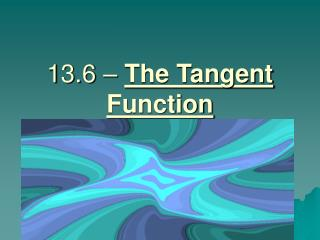Download Presentation13.6 – The Tangent Function

# 13.6 – The Tangent Function - PowerPoint PPT PresentationDownload Presentation## 13.6 – The Tangent Function

- - - - - - - - - - - - - - - - - - - - - - - - - - - E N D - - - - - - - - - - - - - - - - - - - - - - - - - - -
##### Presentation Transcript

1. 13.6 – The Tangent Function

2. I. Graphing the Tangent Function • The tangent of an angle is derived from the coordinates of a point on a line that is tangent to a circle. • Tangent θ – the y – coordinate of the point where the line containing the terminal side of the angle intersects the tangent line x = 1.

3. THINK: TANGENT = OPPOSITE/ADJACENT • Therefore, the tangent is equal to sin/cos

4. Notice as you graph the tangent function it, approaches infinity, and thus has asymptotes at specified values.

5. II. Properties of y = a tan b θ • There is no amplitude • Once cycle occurs in the interval from (-π/2b) to (π/b) π/b is the period of the function there is vertical asymptotes at each end of the cycle

6. Example 1: graph the following: • A) y = 50 tan θ • B) f(x) = tan (π/6)θ

7. Example 2: solve the following: • A) tan θ = 2 • B) 6 tan θ = 1